# Convert an equation to Python

• Python
I have several equations and need to convert it into Python. The problem is that I tried to plot a graph according to the equation. However, the graph that I get is not the same as the original one.

In the paper, the equation of error probability for MIM attack is given by:

First Image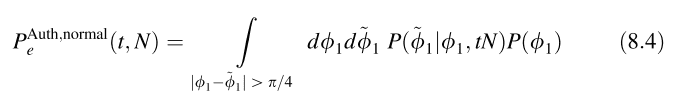Second Image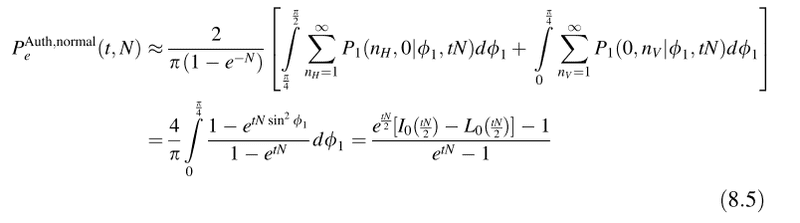The equation to calculate the error probability of PNS attack is given by: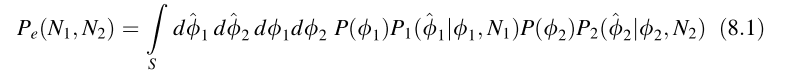Where the region condition satisfied: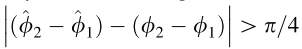The error probability of PNS attack should be plotted like this: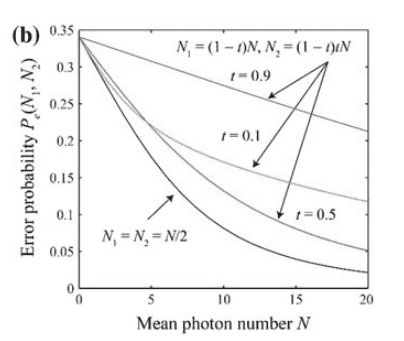My question: How to insert equation 8.1 into equation 8.5?

This is my python code according to equation 8.5:

Python:
import matplotlib.pyplot as plt
import math
import numpy as np
from scipy.special import iv,modstruve

x=[0, 5, 10, 15, 20]
t= 0.9
x = np.array(x)
y = (np.exp(x*t/2)*(iv(0, x*t/2) - modstruve(0,x*t/2))-1)/(np.exp(x*t/2-1))

plt.plot(x, y, label='Normal')
plt.xlabel('Mean photon number N')
plt.ylabel('Error probabiity')
plt.scatter(x,y)
plt.title('N/2')
plt.ylim([0, 0.5])
plt.legend()
plt.show()

Thank you.

#### Attachments

Mentor
When x=0 then N=0 hence the denominator is zero and so you are dividing zero according to the equation.

In your code, you misplaced the last paren. it should look like this:

Python:
y = (   np.exp(x*t/2) * (iv(0,x*t/2) - modstruve(0,x*t/2)) - 1   )  /  (np.exp(x*t/2) - 1)

This code now gives a runtime divide error at zero but the curve looks more like what you want.